Section A

# Graph based KNN for Optimizing Index of News Articles

Taeho Jo *
*Corresponding Author: Taeho Jo, Hongik University, 94 Wausan-ro Mapo-gu Seoul, 04066, Korea, +82-2-320-1114, tjo018@hongik.ac.kr

© Copyright 2016 Korea Multimedia Society. This is an Open-Access article distributed under the terms of the Creative Commons Attribution Non Commercial License (http://creativecommons.org/licenses/by-nc/4.0/) which permits unrestricted non-commercial use, distribution, and reproduction in any medium, provided the original work is properly cited.

Received: Aug 12, 2016 ; Revised: Sep 09, 2016 ; Accepted: Oct 10, 2016

Published Online: Sep 30, 2016

## Abstract

This research proposes the index optimization as a classification task and application of the graph based KNN. We need the index optimization as an important task for maximizing the information retrieval performance. And we try to solve the problems in encoding words into numerical vectors, such as huge dimensionality and sparse distribution, by encoding them into graphs as the alternative representations to numerical vectors. In this research, the index optimization is viewed as a classification task, the similarity measure between graphs is defined, and the KNN is modified into the graph based version based on the similarity measure, and it is applied to the index optimization task. As the benefits from this research, by modifying the KNN so, we expect the improvement of classification performance, more graphical representations of words which is inherent in graphs, the ability to trace more easily results from classifying words. In this research, we will validate empirically the proposed version in optimizing index on the two text collections: NewsPage.com and 20NewsGroups.

Keywords: Graph based KNN; Graph Similarity; Index Optimization

## I. INTRODUCTION

Index optimization refers to the process of optimizing a list of words which indicates a text for maximizing the information retrieval efficiency and performance. In the index optimization task, important words should be expanded by adding their semantically similar words for improving the reliability and unimportant ones should be removed for improving the efficiency. The scope of this research is restricted to the classification task where each word is classified into one of the three classes: ‘important word’ as the target of expansion, ‘neutral word’ as only inclusion, and ‘unimportant word’ as target of removal. We prepare the sample words which are labeled with one of the three classes and construct the classification capacity by learning them. In this research, we assume that the supervised learning algorithms are used as the approach to the task.

Let us consider the facts which provide the motivations for doing this research. Requiring many features for encoding words or texts into numerical vectors causes a large amount of computation time . The sparse distribution in each numerical vector as results from using too many features caused very poor discriminations . Recently, it is very popular trend to represent knowledge into ontologies as the graphs . Therefore, in this research, we attempt to encode words into graphs and modify the KNN (K Nearest Neighbor) into its graph based version, motivated by the above facts.

Let us mention some ideas as the proposal of this research. In this research, we encode each word into a graph with its vertices which indicate text identifiers and its edges which indicate the semantic relations between them. The index optimization is viewed into the task of classifying words into one of the three categories and the similarity measure between two graphs is defined. The KNN is modified into the graph based version where a graph is given as the input data by itself, based on the similarity measure, and it is used as the approach to the index optimization. However, we need the corpus which provides the context for representing words into graphs.

We also consider the benefits which are provided by this research as some points. We may expect the more symbolic and graphical representations of words as indicated inherently by graphs. The improved discrimination is expected by avoiding the sparse distributions which appear frequently in numerical vectors which represent words. We expect the better performance by representing words into alternative structured forms to numerical vectors; the problems which are caused by encoding words into numerical vectors are solved, completely. Hence, the goal of this research is to implement the index optimization system with the benefits as a module of the information retrieval systems.

This article is organized into the four sections. In Section II, we survey the relevant previous works. In Section III, we describe in detail what we propose in this research. In Section IV, we validate empirically the performance of the proposed version of KNN on the two text collections: NewsPage.com and 20NewsGroups. In Section V, we mention the remaining tasks for doing the further research.

## II. PREVIOUS WORKS

In this section, we survey the previous cases of encoding texts into structured forms for using the machine learning algorithms to text mining tasks. The three main problems, huge dimensionality, sparse distribution, and poor transparency, have existed inherently in encoding texts into numerical vectors. In previous works, various schemes of preprocessing texts have been proposed, in order to solve the problems. In this survey, we focus on the process of encoding texts into alternative structured forms to numerical vectors. In other words, this section is intended to explore previous works on solutions to the problems.

Now, we mention the popularity of encoding texts into numerical vectors, and the proposal and the application of string kernels as the solution to the above problems. In 2002, Sebastiani insisted that the numerical vectors are the standard representations of texts in applying the machine learning algorithms to the text classifications . In 2002, Lodhi et al. proposed the string kernel as a kernel function of raw texts in using the SVM (Support Vector Machine) to the text classification . In 2004, Lesile et al. used the version of SVM which proposed by Lodhi et al. to the protein classification . In 2004, Kate and Mooney also used the SVM version for classifying sentences by their meanings .

It was proposed that texts are encoded into tables instead of numerical vectors, as the solutions to the above problems: huge dimensionality, sparse distribution, and poor transparency. In 2008, Jo and Cho proposed the table matching algorithm as the approach to text classification . In 2008, Jo also applied his proposed approach to the text clustering, as well as the text categorization . In 2011, Jo described as the technique of automatic text classification in his patent document . In 2015, Jo improved the table matching algorithm into its more stable version .

Previously, it was proposed that texts should be encoded into string vectors as other structured forms. In 2008, Jo modified the k means algorithm into the version which processes string vectors as the approach to the text clustering . In 2010, Jo modified the two supervised learning algorithms, the KNN and the SVM, into the version as the improved approaches to the text classification . In 2010, Jo proposed the unsupervised neural networks, called Neural Text Self Organizer, which receives the string vector as its input data . In 2010, Jo applied the supervised neural networks, called Neural Text Categorizer, which gets a string vector as its input, as the approach to the text classification .

The above previous works proposed the string kernel as the kernel function of raw texts in the SVM, and tables and string vectors as representations of texts, in order to solve the problems. Because the string kernel takes a large amount of computation time to compute their values, it was used for processing short strings or sentences rather than texts. In the previous works on encoding texts into tables, only table matching algorithm was proposed; there is no attempt to modify the machine algorithms into their table based version. In the previous works on encoding texts into string vectors, only frequency was considered for defining features of string vectors. Words which are used as features of numerical vectors which represent texts have their semantic similarities among them, so the similarities will be used for processing sparse numerical vectors, in this research.

## III. PROPOSED WORK

This section is concerned with encoding words into graphs, modifying the KNN (K Nearest Neighbor) into the graph based version and applying it to the index optimization, and consists of the four sections. In Section 3.1, we deal with the process of encoding words into graphs. In Section 3.2, we describe formally the process of computing the similarity between two graphs. In Section 3.3, we do the graph vector based KNN version as the approach to the index optimization. In Section 3.4, we focus on the process of applying the KNN to the given task with viewing it into a classification task.

3.1. Word Encoding

This section is concerned with the process of encoding a word into a graph as illustrated in Figure 1. A graph is defined into two sets: the vertex set and the edge set. The vertices and edges correspond to text identifiers and their relationships, respectively. A word is represented by a weighted and undirected graph.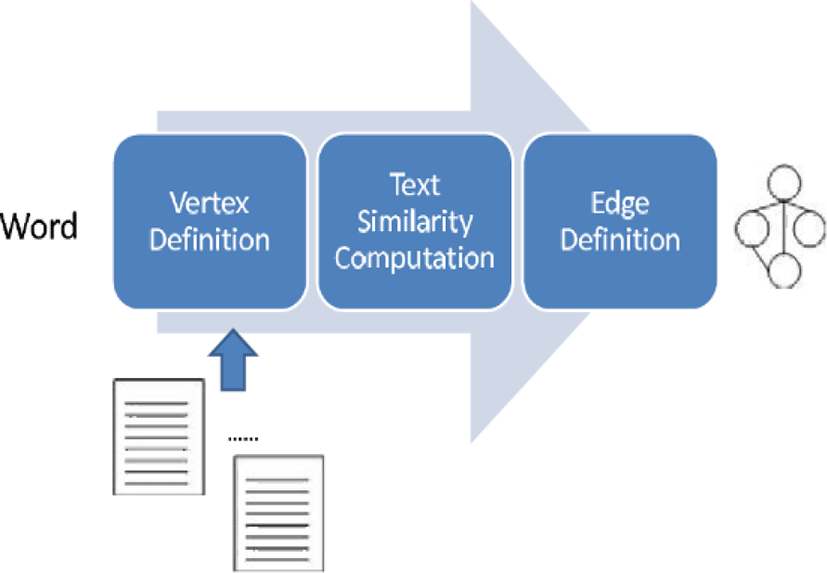Fig. 1. Steps of Encoding Word into Graph.

Before encoding a word into its own graph, we need to construct an inverted index where each word is linked to a list of text. A list of words may be generated by indexing a corpus. Each word is associated with a list of texts which include it. In texts, each word has its own weight, posting information, and statistical information.

Each vertex may be defined from the inverted index. In encoding a word into a graph, vertices correspond to list of texts which are linked to the given word. Some of them are selected by their weights as vertices; they are notated as follows:

${W}_{i}\left(v\right)=\left\{{v}_{i1},{v}_{i2},...,{v}_{in}\right\}$

We consider the ranked selection where a fixed number of texts are selected by ranking them and the score based one where the texts whose weights are greater than or equal to a threshold are selected as the selection schemes. From the inverted index, we extract a set of vertices which indicate text identifiers.

We need to define the set of edges as well as that of vertices for representing a word into a graph. We compute similarities of all possible pairs of vertices which indicate texts. We construct the similarity matrix whose entries indicates similarity measures among texts from a corpus. We select text pairs whose similarities are more than the given threshold, and define the set which consists of edges as follows:

${W}_{i}\left(e\right)=\left\{{e}_{i1},{e}_{i2},...,{e}_{ip}\right\}$

The process of building the similarity matrix and computing the similarity between texts will be described in section 3.2.1.

Let us consider how to represent a graph into its structured form in the implementation level. We may mention the adjacency matrix where vertices correspond to its rows and columns and entries indicate the edge weights. We regard the linked list where vertices are given as nodes and edges are given as pointers between them as another representation of a graph. A graph is represented into a list of edges which are given as pairs of vertex identifiers and each weight is associated with its own weight. In this research, we adopt the third scheme where a graph is represented into a set of edges.

3.2. Graph

This section is concerned with the operation of graphs and the basis for carrying it out. It consists of two subsections and assumes that a corpus is required for performing the operation. In Section 3.2.1, we describe the process of constructing the similarity matrix from a corpus. In Section 3.2.2, we define the operation on graphs mathematically. Therefore, this section is intended to describe the similarity matrix and the operation on them.

3.2.1. Similarity Matrix

This subsection is concerned with the similarity matrix as the basis for performing the semantic operation on string vectors. Each row and column of the similarity matrix corresponds to a text in the corpus. The similarities of all possible pairs of texts are given as normalized values between zero and one. The similarity matrix which we construct from the corpus is the N X N square matrix with symmetry elements and 1’s diagonal elements.

Each entry of the similarity matrix indicates a similarity between two corresponding texts. The two documents, di, and dj are indexed into two sets of words, Di, and Dj. The similarity between the two texts is computed by equation (1),

$sim\left({d}_{i},{d}_{j}\right)=\frac{2|{D}_{i}{\cap }^{\text{​}}{D}_{j}|}{|{D}_{i}|+|{D}_{j}|},$
(1)

where |Di| is the cardinality of the set, Di. The similarity is always given as a normalized value between zero and one; if two documents are exactly same to each other, the similarity becomes 1.0 as follows:

$sim\left({d}_{i},{d}_{i}\right)=\frac{2|{D}_{i}\cap {D}_{i}|}{|{D}_{i}|+|{D}_{j}|}=1.0$

and if two documents have no shared words, DiDj = Ø, the similarity becomes 0.0 as follows:

$sim\left({d}_{i},{d}_{i}\right)=\frac{2|{D}_{i}\cap {D}_{i}|}{|{D}_{i}|+|{D}_{j}|}=0.0$

The more advanced schemes of computing the similarity will be considered in next research.

From the text collection, we build N X N square matrix as follows:

$\left[\begin{array}{cccc}{s}_{11}& {s}_{12}& ...& {s}_{1N}\\ {s}_{21}& s{}_{22}& ...& {s}_{2N}\\ ...& ...& ...& ...\\ {s}_{N1}& {s}_{N2}& ...& {s}_{NN}\end{array}\right]$

N individual texts which are contained in the collection correspond to the rows and columns of the matrix. The entry, Sij is computed by equation (1) as follows:

${s}_{ij}=sim\left({d}_{i},{d}_{j}\right)$

The overestimation or underestimation of text lengths are prevented by the denominator in equation (1). To the number of texts, N, it costs quadratic complexity,O(N2), to build the above matrix

Let us characterize the above similarity matrix, mathematically. Because each column and row corresponds to its same text in the diagonal positions of the matrix, the diagonal elements are always given 1.0 by equation (2). In the off-diagonal positions of the matrix, the values are always given as normalized ones between zero and one, because of 0≤2|DiDi|≤|Di|+|Dj| from equation (2). It is proved that the similarity matrix is symmetric, as follows:

${s}_{ij}=sim\left({d}_{i},{d}_{j}\right)=\frac{2|{D}_{i}{\cap }^{\text{​}}{D}_{j}|}{|{D}_{i}|+|{D}_{j}|}=\frac{2|{D}_{j}{\cap }^{\text{​}}{D}_{i}|}{|{D}_{j}|+|{D}_{i}|}=sim\left({d}_{j},{d}_{i}\right)={s}_{ji}$

Therefore, the matrix is characterized as the symmetry matrix which consists of the normalized values between zero and one.

The similarity matrix may be constructed automatically from a corpus. The N texts which are contained in the corpus are given as the input and each of them is indexed into a list of words. All possible pairs of texts are generated and the similarities among them are computed by equation (1). By computing them, we construct the square matrix which consists of the similarities. Once making the similarity matrix, it will be used continually as the basis for performing the operation on string vectors.

3.2.2. Similarity between Graphs

This section is concerned with the scheme of computing a similarity between two graphs. Words are encoded into graphs where vertices are text identifiers and edges are the similarity between texts. We assume that each graph is a set of edges and consider the three cases for computing the similarity between graphs: both coincidence, either coincidence, and no coincidence. The similarity between graphs is computed by averaging similarities among edges and is always given as a normalized value between zero and one.

We need to consider the similarity between two individual edges, ei and ej which is notated by sim(ei,ej), and each weighted edge consist of two nodes and its weight as follows:

${e}_{i}=\left({v}_{k},{v}_{l},{w}_{i}\right)$

and the edge weight is notated by w(ei) = wi. If no node is shared by two edges like (A,B,0.2) and (C,D,0.4), the similarity becomes zero. If only one node is shared by two edges like (A,B,0.2) and (B,C,0.4), the similarity becomes the product of two weights as follows:

$sim\left({e}_{i},{e}_{j}\right)=w\left({e}_{i}\right)w\left({e}_{j}\right)$

If both nodes are shared, the similarity becomes the average of the two weights as follows:

$sim\left({e}_{i},{e}_{j}\right)=\frac{1}{2}\left(w\left({e}_{i}\right)+w\left({e}_{j}\right)\right).$

It is assumed that each weight between edges is always given as normalized value between zero and one.

The two graphs, G1 and G2, are expressed into the two sets:

${G}_{1}=\left\{{e}_{11},{e}_{12},..,{e}_{1n}\right\}\text{\hspace{0.17em}}\text{\hspace{0.17em}}\text{and}\text{​}\text{​}\text{\hspace{0.17em}}\text{\hspace{0.17em}}{G}_{2}=\left\{{e}_{21},{e}_{22},..,{e}_{2n}\right\},$

and it is assumed that both graphs have same number of edges. All possible pairs of edges are generated from the two graphs. For each edge in one graph, its similarities with the edges in the other are computed, and the maximum among them is obtained as the similarity between an edge and a graph, by equation (2).

$sim\left({e}_{1i},{G}_{2}\right)=\underset{k=1}{\overset{n}{\mathrm{max}}}sim\left({e}_{1i},{e}_{2k}\right)$
(2)

The similarity between the two graphs is set by averaging over the maximum similarities of edges with the other by equation (3),

$sim\left({G}_{1},{G}_{2}\right)=\frac{1}{n}\sum _{i=1}^{n}sim\left({e}_{1i},{G}_{2}\right)$
(3)

Because the weights of edges are always given as normalized values, the similarity between graphs is always so.

Let us characterize the operation for computing the similarity between graphs, mathematically. If the two graphs, G1 and G2 are identical to each other and all edges are weighted with 1.0 values, w(ei) = 1.0, ∀i, the similarity between the two graphs becomes 1.0.

$\begin{array}{c}sim\left({e}_{i},{e}_{i}\right)=\frac{1}{2}\left(w\left({e}_{i}\right)+w\left({e}_{i}\right)\right)=1.0\\ sim\left({G}_{1},{G}_{2}\right)=\frac{1}{n}\sum _{i=1}^{n}sim\left({e}_{i},{e}_{i}\right)=1.0\\ sim\left({G}_{1},{G}_{2}\right)=1.0\end{array}$

If the two graphs, G1 and G2 are so with different weights, the similarity between the two graphs is the average over weights of two graphs as follows:

$\begin{array}{l}sim\left({e}_{1i},{e}_{2i}\right)=\frac{1}{2}\left(w\left({e}_{i}\right)+w\left({{e}^{\prime }}_{i}\right)\right)\\ sim\left({G}_{1},{G}_{2}\right)=\frac{1}{n}\sum _{i=1}^{n}sim\left({e}_{i},{{e}^{\prime }}_{i}\right)\\ =\frac{1}{2n}\sum _{i=1}^{n}\left(w\left({e}_{i}\right)+w\left({{e}^{\prime }}_{i}\right)\right)\\ =\frac{1}{2n}\left(\sum _{i=1}^{n}w\left({e}_{i}\right)+\sum _{i=1}^{n}w\left({{e}^{\prime }}_{i}\right)\right)\end{array}$

If there is no shared edge between the two graphs, the similarity becomes zero as follows:

$\begin{array}{l}sim\left({e}_{1i},{G}_{2}\right)=\underset{k=1}{\overset{n}{\mathrm{max}}}sim\left({e}_{1i},{e}_{2k}\right)=0.0\\ sim\left({G}_{1},{G}_{2}\right)=\frac{1}{n}\sum _{i=1}^{n}sim\left({e}_{1i},{G}_{2}\right)=\frac{1}{n}\sum _{i=1}^{n}0.0=0.0\end{array}$

The similarity between the two graphs is always a normalized value between zero and one as proved from the mathematical characterization.

Let us consider the complexity of computing a similarity between graphs to the number of edges in both graphs. The number of all possible pairs becomes $\frac{n\left(n-1\right)}{2}$ to the number of edge, n. The similarities of all possible pairs are computed by the above process, $\frac{n\left(n-1\right)}{2}$ times. We derive the quadratic complexity O(n2) , for computing the similarities. Therefore, we need to optimize the number of edges for representing a word into a graph by controlling the threshold between the reliability and the computation speed.

3.3. Proposed Version of KNN

This section is concerned with the proposed KNN version as the approach to the text categorization. Raw texts are encoded into graphs by the process which was described in Section 3.1. In this section, we attempt to the traditional KNN into the version where a graph is given as the input data. The version is intended to improve the classification performance by avoiding problems from encoding texts into numerical vectors.

The traditional KNN version is illustrated in Figure 2. The sample words which are labeled with the positive class or the negative class are encoded into numerical vectors. The similarities of the numerical vector which represents a novice word with those representing sample words are computed using the Euclidean distance or the cosine similarity. The k most similar sample words are selected as the k nearest neighbors and the label of the novice entity is decided by the majority of their labels. However, note that the traditional KNN version is very fragile in computing the similarity between very sparse numerical vectors.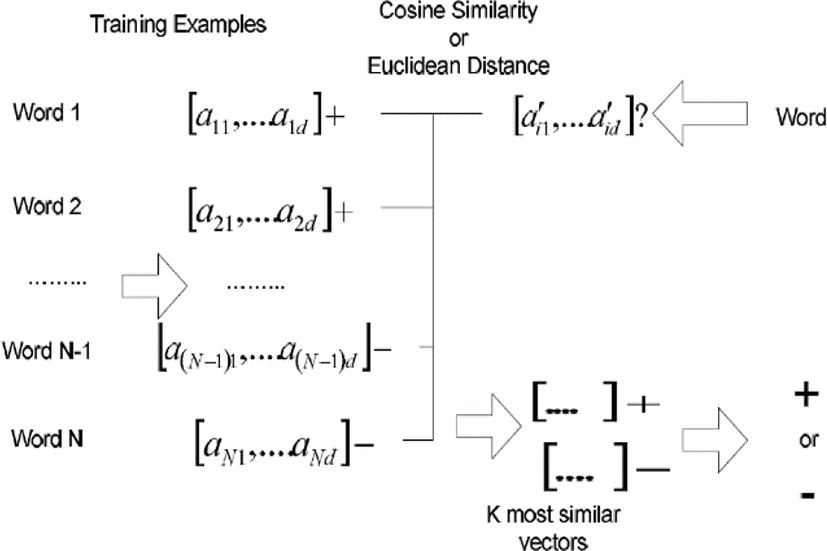Fig. 2. The Traditional Version of KNN

Separately from the traditional one, we illustrate the classification process by the proposed version in Figure 3. The sample texts labeled with the positive or negative class are encoded into graphs by the process described in section 1. The similarity between two graphs is computed by the scheme which was described in Section 3.2.2. Identically to the traditional version, in the proposed version, the k most similarity samples are selected, and the label of the novice one is decided by voting ones of sample entities. Because the sparse distributions in graphs are never available inherently, the poor discriminations by sparse distributions are certainly overcome in this research.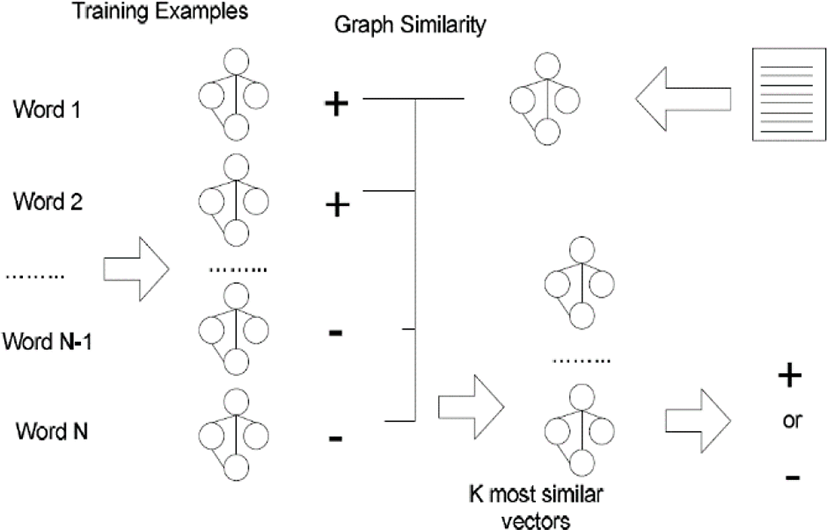Fig. 3. The Proposed Version of KNN

We may derive some variants from the proposed KNN version. We may assign different weights to selected neighbors instead of identical ones: the highest weights to the first nearest neighbor and the lowest weight to the last one. Instead of a fixed number of nearest neighbors, we select any number of training examples within a hyper-sphere whose center is the given novice example as neighbors. The categorical scores are computed proportionally to similarities with training examples, instead of selecting nearest neighbors. We may also consider the variants where more than two variants are combined with each other.

In this research, once we define the similarity measure between graphs, we are able to modify the KNN. For modifying the k means algorithm, we need to define one more operation which build prototype graph as representative among ones. For modifying the perceptron or the MLP (Multiple Layer Peceptron) where both input and weights are given as graphs, we need the update rule of graphs. In order to define more advanced operations on graphs, we need to do more theoretical research on operations based on the graph theory.

3.4. Application to Index Optimization

This section is concerned with the scheme of applying the proposed KNN version which was described in section 3 to the index optimization task. Before doing so, we need to transform the task into one where machine learning algorithms are applicable as the flexible and adaptive models. We prepare the words which are labeled with ‘expansion’, ‘inclusion’ or ‘removal’ as the sample data. The words are encoded into tables by the scheme which was described in Section 3.2.

In this research, the index optimization is viewed as a classification task, as shown in Figure 4. A text is given as the input, and a list of words is extracted by indexing the text. Each word is classified by the classifier into one of the three categories: ‘expansion’, ‘inclusion’, or, ‘removal’. In the task, the text is mapped into words which are classified with ‘expansion’ or ‘inclusion’. The similar words to one labeled with ‘expansion’ will be added from external sources.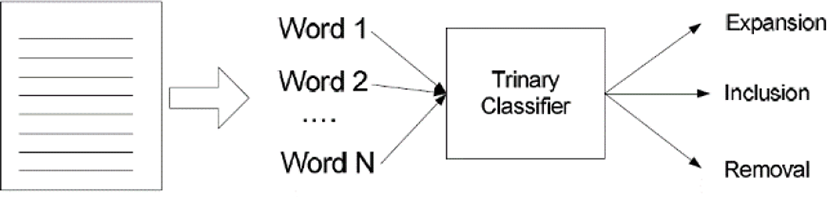Fig. 4. Map of Index Optimization into Classification Task

We need to prepare sample words which are labeled with one of the three categories, before classifying a novice one or ones. A text collection is segmented into sub-collections of content based similar words which are called domains, manually or automatically. We prepare sample words which are labeled manually, domain by domain. To each domain, we assign and train a classifier with the words in the corresponding sub-collection. When a text is given as the input, the classifier which corresponds to the most similar domain is selected among them.

Let us consider the process presented in Figure 5 where an article is given as the input and a list of essential words is extracted as the output. We nominate the classifier which corresponds to the subgroup which is closest to the given article with respect to its content. A list of words is extracted by indexing the article, and each word is encoded. The words are classified by the nominated classifier into one of the three categories, and we select ones which are labeled with ‘expansion’ or ‘reservation’ as the optimized index. The addition of external words which are semantically similar as ones labeled with ‘expansion’ is set as the subsequent task.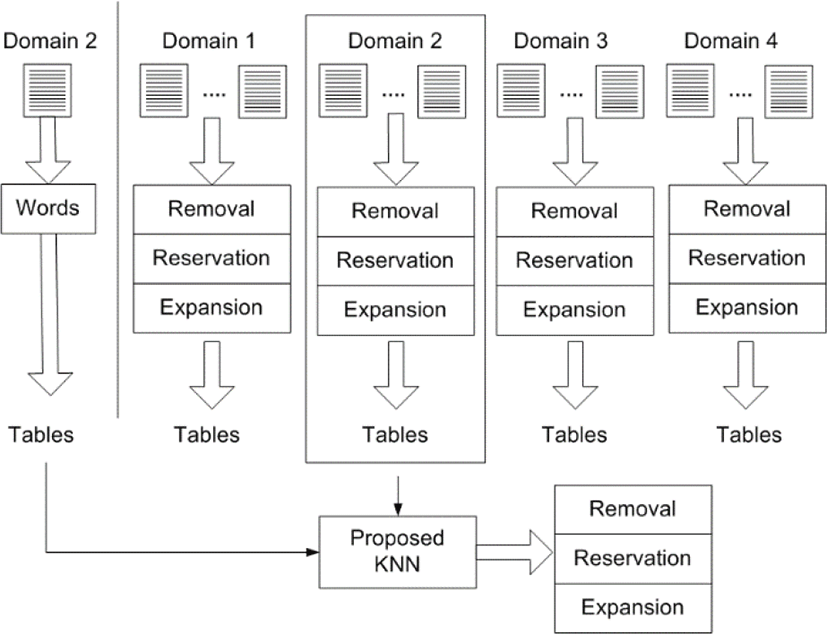Fig. 5. Process of applying KNN to Index Optimization

Even if the index optimization is viewed as an instance of word categorization, it needs to be distinguished from the topic based word categorization. The word categorization is given as a single multiple classification or multiple binary classifications, whereas the index optimization is done as a multiple classification or three binary classification tasks. In the word categorization, each word is classified semantically into one or some of the predefined topics, whereas in the index optimization, it is classified one of the three actions. In the word categorization, each word is classified by its meaning, whereas in the index optimization, it is classified by its importance to the given text. In the word categorization, when the given task is decomposed into binary classification tasks, a classifier is assigned to each topic, whereas, in the index optimization, a classifier is done to each domain.

## IV. EXPERIMENTS

This section is concerned with the empirical validations of the proposed version of KNN, as the approach to index optimization. The goal of these experiments is to compare the proposed version where words are encoded into graphs, with the traditional one where they are done into numerical vectors. In Section 1, we make the empirical validations of the proposed version about the index optimization within each of four topics from the text collection, NewsPage.com. In Section 2, we make the empirical validation in each topic from another collection, 20NewsGroups.

4.1. NewsPage.com

This section is concerned with the experiments for evaluating the two versions of KNN as the index optimization tools within each topic from NewsPage.com. NewsPage.com from which the experimental data is generated, is the small collection of texts which were previously used for evaluating text classification algorithms. Words are extracted by indexing included texts and the test data is constructed by labeling them with one of the three importance levels: expansion, inclusion, and removal. The results from evaluating the two versions based on the labeled words are presented in Figure 1.

We illustrate the distributions of texts and words which are labeled with one of the three importance levels in the collection, NewsPage.com, in Table 1. The text collection, NewsPage.com, is built by copying and pasting news articles in the web site, newspage.com, individually, topic by topic. The text collection was previously used for evaluating performances of text classifiers which decide topics of novice texts, automatically. Currently, we use the text collection to extract words by indexing individual texts and manually labeling word with their own importance level to its belonging text. The test data which is used in this experiment consists of the four collections of words which are labeled with one of the three importance levels, exclusively; in each topic, 125 words are allocated evenly to each importance level, among 375 ones.

Table 1. Distribution of Texts and Labeled Words in NewsPage.com
Category #Texts #Training Words #Test Words
Health 500 300 75
Internet 500 300 75
Sports 500 300 75
Total 2,000 1,200 300

Let us mention the process of doing the empirical validations on the topics from NewsPage.com. Words are extracted from individual texts, and labeled manually by voting the decisions of three subjects. We select 125 words at random in each importance level; we obtain 375 words with their completely balanced distribution over the three importance levels, in each topic. The set of 375 words is divided into the two sets: 300 words are allocated to the training set, and the others, 75 words, are allocated to the test set, as shown in Table 1. The words are encoded into 50 dimensional numerical vectors for evaluating the traditional version, and 50 sized graphs which consists of 50 edges, for evaluating the proposed version.

In Figure 6, we illustrate the results from applying the two versions of KNN to the index optimization on 75 test words in each topic. The y-axis in Figure 6 indicates the accuracy of classifying the 75 test words into one of the three importance levels. The gray bars and the black bars indicate the performances of the traditional version and the proposed version, respectively. The x-axis indicates the list of topics from NewsPage.com and the average over them. As shown in Figure 1, the proposed version of KNN works better than the traditional one over the four topics.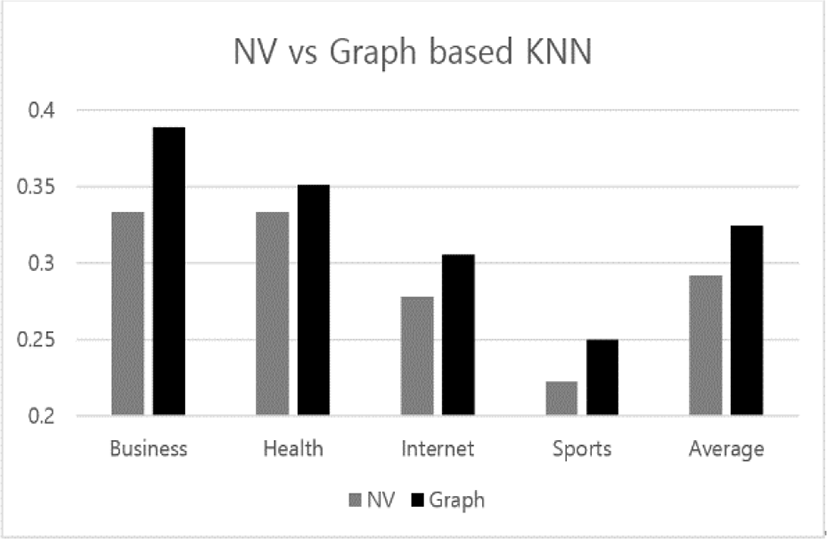Fig. 6. Results from Evaluating NV and Graph based KNN in Topics of NewsPage.com

Let us discuss the results from comparing the two versions with each other in performing the index optimizations on the topics from NewsPage.com. The both versions of KNN works best in the topic, ‘Business’. In average, the traditional version has its classification accuracy below 0.3, while the proposed version does it above the value. Because depending on individual text as well as topic, same word may be labeled differently, the classification performances of the both versions is not high; stay below 0.5. However, the significance of this experiment is that the proposed version works relatively better.

4.2. 20NewsGroups

This section is concerned with the experiments for evaluating the both versions as the index optimizations in the topics from 20NewsGroups. The process of doing the experiments to each topic is the same to that of experiments which was covered in Section 4.1. The proposed of KNN obtains the three wins among the four topics; it works slightly better than traditional one, on average.

In Table 2, we illustrate the distribution of the number of texts and labeled words over the four representative topics within the scientific domain in 20NewsGroups. The text collection, 20NewsGroups, actually consists of the 20 topics; we select the four topics under its parent topic, ‘Science’ among them. We obtain 375 labeled words, keeping the completely balanced distributions over the three importance levels in each topic. Among them, 300 words are used as training words, and 75 words are used as test words for evaluating the both versions. The two text collections which are used in the experiments of this research consist of texts which are labeled with only one category, exclusively, but the text collection, Reuter21578, which has been used most popularly for evaluating text classification algorithms, consists of texts which are labeled with more than one.

Table 2. Distribution of Texts and Labeled Words in Four Representative Topics of 20NewsGroups
Category #Texts #Training Words #Test Words
Electro 1,000 300 75
Medicine 1,000 300 75
Script 1,000 300 75
Space 1,000 300 75
Total 4,000 1,200 300

The process of doing the experiment in each category is the same to identical to that of the experiment in Section 4.1. The words are extracted from texts in the selected topics of 20NewsGroups, and they are labeled by voting the decisions of three subjects. We select 125 words in each importance level at random, so that the completely balanced distribution is maintained. As shown in Table 2, the set of labeled words is divided identically to the case in the previous experiment. The words are encoded into numerical vectors and graphs with the size which is identical to the case of the previous experiment.

Figure 7 presents the results from applying the two versions of KNN to the index optimization on words from the four topics of 20NewsGroups. The result framework in Figure 2 is identical to that in Figure 7. The differences from the results which are shown in Figure 7 are the listed four topics and the accuracy values of the two versions. The gray bar and the black bar in each topic indicate the accuracies of the traditional and proposed versions, respectively. Even if the proposed version is lost in the topic, ‘Electro’, it works slightly better than the traditional one, on average.Fig. 7. Results from Evaluating NV and Graph based KNN in Topics of 20NewsGroup

Let us discuss the results from the experiments which is shown in Figure 7. The both versions of KNN works best in the topic, ‘Med’. The accuracies of the both versions are between 0.34 and 0.36 on average; the proposed version has its slightly higher accuracy. However, the proposed version wins outstandingly over the traditional one, in three of the four topics. Finally, with the view of tournaments of the two versions, it is concluded that the proposed version wins by three to one.

## V. CONCLUSION

Let us consider the significances of this research. The importance of words is divided into the three levels and the index optimization is viewed as a classification. Words are encoded into graphs as the alternative representations to numerical vectors and the similarity measures between them is defined. The KNN is modified into the graph based version which receives a graph as its input data, instead of a numerical vector. The modified version of KNN is applied to the task, index optimization.

Let us mention the remaining tasks for doing the further research. We need to observe the performances of the modified version for optimizing index of texts in specific domains: finance, law, engineering, and medicine. We define more operations on graphs and characterize them mathematically, based on the graph theory. We modify other machine learning algorithms such as Naïve Bayes, Support Vector Machine, Decision Tree, and Neural Networks, like so. We may consider implementing the graph based deep learning algorithms which recently became the popular trends of machine learning algorithms.

## Acknowledgement

This work was supported by 2016 Hongik University Research Fund.

## REFERENCES

.

T. Jo, “The Implementation of Dynamic Document Organization using Text Categorization and Text Clustering,” PhD Dissertation of University of Ottawa, 2006..

N. F. Noy and C. D. Hafner, “State of the Art in Ontology Design,” AI Magazine, vol. 18, no. 3, 1997..

D. Allemang and J. Hendler, Semantic Web for the Working Ontologies, Mrgan Kaufmann, 2011..

F. Sebastiani, “Machine Learning in Automated Text Categorization,” ACM Computing Survey, vol. 34, no. 1, pp. 1-47, 2002..

H. Lodhi, C. Saunders, J. Shawe-Taylor, N. Cristianini and C. Watkins, “Text Classification with String Kernels,” Journal of Machine Learning Research, vol. 2, no. 2, pp. 419-444, 2002..

C. S. Leslie, E. Eskin, A. Cohen, J. Weston, and W. S. Noble, “Mismatch String Kernels for Discriminative Protein Classification,” Bioinformatics, vol. 20, no. 4, pp. 467-476, 2004.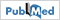.

R. J. Kate and R. J. Mooney, “Using String Kernels for Learning Semantic Parsers,” in Proceedings of the 21st International Conference on Computational Linguistics and the 44th annual meeting of the Association for Computational Linguistics, pp. 913-920, 2006..

T. Jo and D. Cho, “Index based Approach for Text Categorization,” International Journal of Mathematics and Computers in Simulation, vol. 2, no. 1, pp. 127-132, 2008..

T. Jo, “Single Pass Algorithm for Text Clustering by Encoding Documents into Tables,” Journal of Korea Multimedia Society, vol. 11, no. 12, pp. 1749-1757, 2008..

T. Jo, “Device and Method for Categorizing Electronic Document Automatically,” Patent Document, 10-2009-0041272, 10-1071495, 2011..

T. Jo, “Normalized Table Matching Algorithm as Approach to Text Categorization,” Soft Computing, vol. 19, no. 4, pp. 839-849, 2015..

T. Jo, “Inverted Index based Modified Version of K-Means Algorithm for Text Clustering,” Journal of Information Processing Systems, vol. 4, no. 2, pp. 67-76, 2008..

T. Jo, “Representation of Texts into String Vectors for Text Categorization,” Journal of Computing Science and Engineering, vol. 4, no. 2, pp. 110-127, 2010..

T. Jo, “NTSO (Neural Text Self Organizer): A New Neural Network for Text Clustering,” Journal of Network Technology, vol. 1, no. 1, pp. 31-43, 2010..

T. Jo, “NTC (Neural Text Categorizer): Neural Network for Text Categorization,” International Journal of Information Studies, vol. 2, no. 2, pp83-96, 2010.